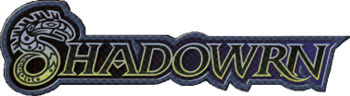Back to the main page

# Mailing List Logs forFrom: Todd Montgomery Just a suggestion about acceleration Fri, 12 Mar 93 17:00:40 CET
[Has nothing to do with determining stats of a vehicle]

Here is a suggestions for acceleration.

This is quick and dirty so bare with me.

In a fighter game a friend of mine is developing for Star Wars,
Acceleration is figured like so,

You have a Maneuver rating, a Speed rating, and a Turn number.
The turn number is Speed Rating / Maneuver Rating (round up).
You can change speed by your turn number. So example.
Maneuver of 3, Speed of 8, Turn of 3 for round 1.
Maneuver of 3, Speed of 5, Turn of 2 for round 2.
or
Maneuver of 3, Speed of 10, Trun of 4 for round 2.

That is the basic concept. Even though this deals with turning of
a starfighter.

Would something like this work for acceleration. Make acceleration
dependent on current speed, and handling. An example such as,

Car with handling of 2, Speed of 190/120. Just for argument.
The car could accelerate by say (190-120)/(2) = + 35 m/ct when cruising.
Or (190-190)/(2) = + 0 m/ct when going 190.
Or (190-10)/(2) = + 90 m/ct when going 10.

This does provide a quick method of determining acceleration.
And it mimicks a non-linear equation. Not differential but close enough.

Take max speed - current speed and then divide by handling. This is
the speed increase amount that is possible.

For deceleration.

Take current Speed / handling is the max amount of max deceleration.

Most cars can stop a lot quicker than they can accelerate, I assume.

Now what in the world does this have to do with vehicle construction?
Honestly, not much! It was just an idea. But it could be of use in
making the play a little more realistic.

-- Quiktek
a.k.a. Todd Montgomery
tmont@****.wvu.wvnet.edu